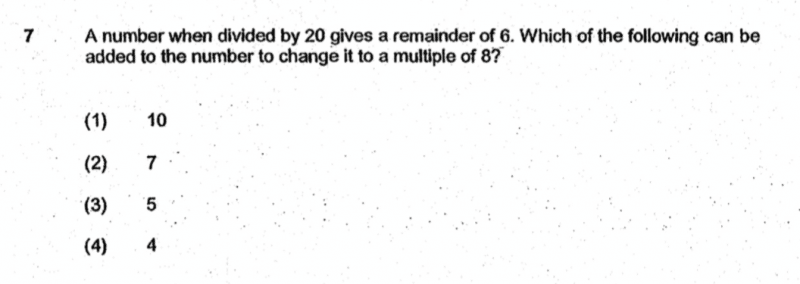# QuestionSource: Henry Park Primary

Numbers which satisfied the first statement were 26, 46, 66, 86,…

Answers (2) & (3) can be eliminated immediately because adding 7 or 5 to the above list will make the number an odd number and thus not divisible by 8.

Adding 4 to the above list of numbers will give you a number ending with 0. The only multiples of 8 ending with 0 are multiples of 40. Again, looking at the list, it is not possible to get 40, 80, 120, etc by adding 4.

So only possible answer is 10, and we can see that

46 + 10 = 56 which is divisible by 8

86 + 10 = 96 which is divisible by 8

Understandably, one might argue that it is not true that 26 + 10 is divisible by 8.

Look closely at the question and note that it just said A number, and not ANY or ALL. So you just need to find one instance that satisfies both conditions.